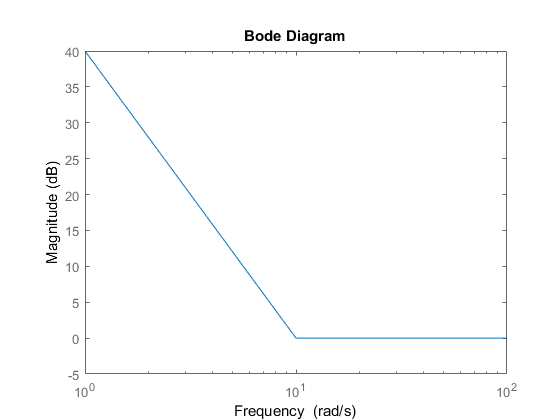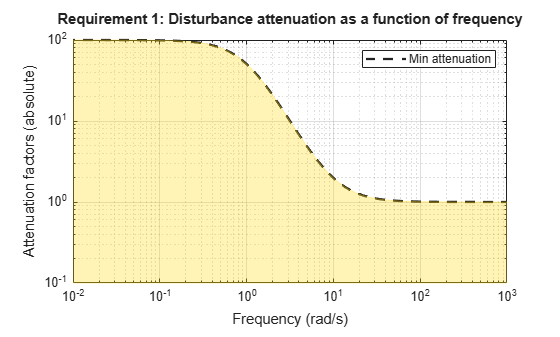# TuningGoal.Rejection class

Package: TuningGoal

Disturbance rejection requirement for control system tuning

## Description

Use `TuningGoal.Rejection` to specify the minimum attenuation of a disturbance injected at a specified location in a control system. This tuning goal helps you tune control systems with tuning commands such as `systune` or `looptune`.

When you use `TuningGoal.Rejection`, the software attempts to tune the system so that the attenuation of a disturbance at the specified location exceeds the minimum attenuation factor you specify. This attenuation factor is the ratio between the open- and closed-loop sensitivities to the disturbance and is a function of frequency. You can achieve disturbance attenuation only inside the control bandwidth. The loop gain must be larger than one for the disturbance to be attenuated (attenuation factor > 1).

## Construction

```Req = TuningGoal.Rejection(distloc,attfact)``` creates a tuning goal for rejecting a disturbance entering at `distloc`. This tuning goal constrains the minimum disturbance attenuation factor to the frequency-dependent value, `attfact`.

### Input Arguments

 `distloc` Disturbance location, specified as a character vector or, for multiple-input tuning goals, a cell array of character vectors. If you are using the tuning goal to tune a Simulink® model of a control system, then `distloc` can include any signal identified as an analysis point in an `slTuner` (Simulink Control Design) interface associated with the Simulink model. Use `addPoint` (Simulink Control Design) to add analysis points to the `slTuner` interface. Use `getPoints` (Simulink Control Design) to get the list of analysis points available in an `slTuner` interface to your model. For example, suppose that the `slTuner` interface contains analysis points `u1` and `u2`. Use `'u1'` to designate that point as the disturbance input when creating tuning goals. Use `{'u1','u2'}` to designate a two-channel disturbance input.If you are using the tuning goal to tune a generalized state-space model (`genss`) of a control system, then `inputname` can include any `AnalysisPoint` channel in the model. For example, if you are tuning a control system model, `T`, which contains an `AnalysisPoint` block with a location named `AP_u`, then `distloc` can include `'AP_u'`. (Use `getPoints` to get a list of analysis points available in a `genss` model.) The constrained disturbance location is injected at the implied input associated with the analysis point, and measured at the implied output:`attfact` Attenuation factor as a function of frequency, specified as a numeric LTI model. `TuningGoal.Rejection` constrains the minimum disturbance attenuation to the frequency-dependent value `attfact`. You can specify `attfact` as a smooth transfer function (`tf` , `zpk`, or `ss` model). Alternatively, you can specify a piecewise gain profile using a `frd` model. For example, the following code specifies an attenuation factor of 100 (40 dB) below 1 rad/s, gradually dropping to 1 (0 dB) past 10 rad/s, for a disturbance injected at `u`. ```attfact = frd([100 100 1 1],[0 1 10 100]); Req = TuningGoal.Rejection('u',attfact); bodemag(attfact) ylim([-5,40])```When you use an `frd` model to specify `attfact`, the gain profile is automatically mapped onto a `zpk` model. The magnitude of this `zpk` model approximates the desired gain profile. Use `viewGoal(Req)` to visualize the resulting attenuation profile. If you are tuning in discrete time (that is, using a `genss` model or `slTuner` interface with nonzero `Ts`), you can specify `attfact` as a discrete-time model with the same `Ts`. If you specify `attfact` in continuous time, the tuning software discretizes it. Specifying the attenuation profile in discrete time gives you more control over the profile near the Nyquist frequency.

## Properties

 `MinAttenuation` Minimum disturbance attenuation as a function of frequency, expressed as a SISO `zpk` model. The software automatically maps the `attfact` input argument to a `zpk` model. The magnitude of this `zpk` model approximates the desired attenuation factor and is stored in the `MinAttenuation` property. Use `viewGoal(Req)` to plot the magnitude of `MinAttenuation`. `Focus` Frequency band in which tuning goal is enforced, specified as a row vector of the form `[min,max]`. Set the `Focus` property to limit enforcement of the tuning goal to a particular frequency band. Express this value in the frequency units of the control system model you are tuning (rad/`TimeUnit`). For example, suppose `Req` is a tuning goal that you want to apply only between 1 and 100 rad/s. To restrict the tuning goal to this band, use the following command:`Req.Focus = [1,100];` Default: `[0,Inf]` for continuous time; `[0,pi/Ts]` for discrete time, where `Ts` is the model sample time. `LoopScaling` Toggle for automatically scaling loop signals, specified as `'on'` or `'off'`. For multiloop or MIMO disturbance rejection tuning goals, the feedback channels are automatically rescaled to equalize the off-diagonal (loop interaction) terms in the open-loop transfer function. Set `LoopScaling` to `'off'` to disable such scaling and shape the unscaled open-loop response. Default: `'on'` `Location` Location of disturbance, specified as a cell array of character vectors that identify one or more analysis points in the control system to tune. For example, if ```Location = {'u'}```, the tuning goal evaluates disturbance rejection at an analysis point `'u'`. If `Location = {'u1','u2'}`, the tuning goal evaluates the rejection at based on the MIMO open-loop response measured at analysis points `'u1'` and `'u2'`. The initial value of the `Location` property is set by the `distloc` input argument when you create the tuning goal. `Models` Models to which the tuning goal applies, specified as a vector of indices. Use the `Models` property when tuning an array of control system models with `systune`, to enforce a tuning goal for a subset of models in the array. For example, suppose you want to apply the tuning goal, `Req`, to the second, third, and fourth models in a model array passed to `systune`. To restrict enforcement of the tuning goal, use the following command: `Req.Models = 2:4;` When `Models = NaN`, the tuning goal applies to all models. Default: `NaN` `Openings` Feedback loops to open when evaluating the tuning goal, specified as a cell array of character vectors that identify loop-opening locations. The tuning goal is evaluated against the open-loop configuration created by opening feedback loops at the locations you identify. If you are using the tuning goal to tune a Simulink model of a control system, then `Openings` can include any linear analysis point marked in the model, or any linear analysis point in an `slTuner` (Simulink Control Design) interface associated with the Simulink model. Use `addPoint` (Simulink Control Design) to add analysis points and loop openings to the `slTuner` interface. Use `getPoints` (Simulink Control Design) to get the list of analysis points available in an `slTuner` interface to your model. If you are using the tuning goal to tune a generalized state-space (`genss`) model of a control system, then `Openings` can include any `AnalysisPoint` location in the control system model. Use `getPoints` to get the list of analysis points available in the `genss` model. For example, if `Openings = {'u1','u2'}`, then the tuning goal is evaluated with loops open at analysis points `u1` and `u2`. Default: `{}` `Name` Name of the tuning goal, specified as a character vector. For example, if `Req` is a tuning goal: `Req.Name = 'LoopReq';` Default: `[]`

## Examples

### Constant Minimum Attenuation in Frequency Band

Create a tuning goal that enforces a attenuation of at least a factor of 10 between 0 and 5 rad/s. The tuning goal applies to a disturbance entering a control system at a point identified as `'u'`.

```Req = TuningGoal.Rejection('u',10); Req.Name = 'Rejection spec'; Req.Focus = [0 5]```

### Frequency-Dependent Attenuation Profile

Create a tuning goal that enforces an attenuation factor of at least 100 (40 dB) below 1 rad/s, gradually dropping to 1 (0 dB) past 10 rad/s. The tuning goal applies to a disturbance entering a control system at a point identified as `'u'`.

```attfact = frd([100 100 1 1],[0 1 10 100]); Req = TuningGoal.Rejection('u',attfact);```

These commands use a `frd` model to specify the minimum attenuation profile as a function of frequency. The minimum attenuation of 100 below 1 rad/s, together with the minimum attenuation of 1 at the frequencies of 10 and 100 rad/s, specifies the desired rolloff.

`attfact` is converted into a smooth function of frequency that approximates the piecewise specified profile. Display the gain profile using viewGoal.

`viewGoal(Req)`The shaded region indicates where the tuning goal is violated.

## Tips

• This tuning goal imposes an implicit stability constraint on the closed-loop sensitivity function measured at `Location`, evaluated with loops opened at the points identified in `Openings`. The dynamics affected by this implicit constraint are the stabilized dynamics for this tuning goal. The `MinDecay` and `MaxRadius` options of `systuneOptions` control the bounds on these implicitly constrained dynamics. If the optimization fails to meet the default bounds, or if the default bounds conflict with other requirements, use `systuneOptions` to change these defaults.

## Algorithms

When you tune a control system using a `TuningGoal`, the software converts the tuning goal into a normalized scalar value f(x). In this case, x is the vector of free (tunable) parameters in the control system. The parameter values are adjusted automatically to minimize f(x) or drive f(x) below 1 if the tuning goal is a hard constraint.

For `TuningGoal.Rejection`, f(x) is given by:

`$f\left(x\right)=\underset{\omega \in \text{\hspace{0.17em}}\Omega }{\mathrm{max}}{‖{W}_{S}\left(j\omega \right)S\left(j\omega ,x\right)‖}_{\infty },$`

or its discrete-time equivalent. Here, S(,x) is the closed-loop sensitivity function measured at the disturbance location. Ω is the frequency interval over which the tuning goal is enforced, specified in the `Focus` property. WS is a frequency weighting function derived from the specified attenuation profile. The gains of WS and `MinAttenuation` roughly match for gain values ranging from –20 dB to 60 dB. For numerical reasons, the weighting function levels off outside this range, unless the specified attenuation profile changes slope outside this range. This adjustment is called regularization. Because poles of WS close to s = 0 or s = `Inf` might lead to poor numeric conditioning of the `systune` optimization problem, it is not recommended to specify attenuation profiles with very low-frequency or very high-frequency dynamics.

To obtain WS, use:

`WS = getWeight(Req,Ts)`

where `Req` is the tuning goal, and `Ts` is the sample time at which you are tuning (`Ts = 0` for continuous time). For more information about regularization and its effects, see Visualize Tuning Goals.

## Compatibility Considerations

expand all

Behavior changed in R2016a

Introduced in R2016a